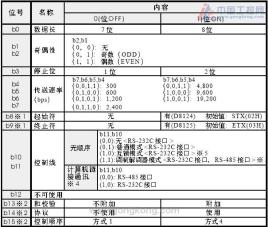Home technology Odd parity

# Odd parity

## Basic information

The parity bit is a binary number that indicates whether the number of 1s in the binary number of a given location number is odd or even. The parity bit is the simplest error detection code. There are two types of parity bits: even parity bits and odd parity bits. If the number of 1s in a given set of data bits is odd, then the even parity bit is set to 1, so that the total number of 1s is even; if the number of 1s in a given set of data bits is even , Then the odd parity bit is set to 1, making the total number of 1s odd.

If odd parity is adopted, an additional bit is added as a check bit when transmitting each byte. The check bit is after the data bit. When the number of "1"s in the actual data is When the number is even, the parity bit is "1", otherwise the parity bit is "0", which ensures that the transmitted data meets the requirements of odd parity. When the receiver receives the data, it will detect the number of "1"s in the data according to the requirements of odd parity. If it is an odd number, it means the transmission is correct, otherwise it means the transmission is wrong.

## Other information

Similarly, the process of even parity is the same as that of odd parity, except that the number of "1"s in the detection data is odd.

As shown in the table below

 7-bit data (1 Number) bytes with check digit Even parity Odd parity digit 0000000(0) td> 0000000 0 0000000 1 1010001(3) 1010001 1 1010001 0 1101001(4) 1101001 0 td> 1101001 1 1111111( 7) 1111111 1 1111111 0

Inference even check: When the number of "1" in the actual data is even, this check The bit is "0", otherwise the parity bit is "1", which ensures that the transmitted data meets the requirements of even parity. When the receiver receives the data, it will detect the number of "1"s in the data according to the requirements of even parity. If it is an even number of "1"s, it means the transmission is correct, otherwise it means the transmission is wrong.

The English abbreviation ODD for odd parity.